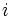# Euler's Formula

Euler's formula is the following identity in complex analysis making use of the imaginary number:## Euler's Identity

The formula can be used to derive an equation known as Euler's identity:This is called the most beautiful equation in mathematics, because it equates the five most common mathematical constants in a perfectly elegant manner.